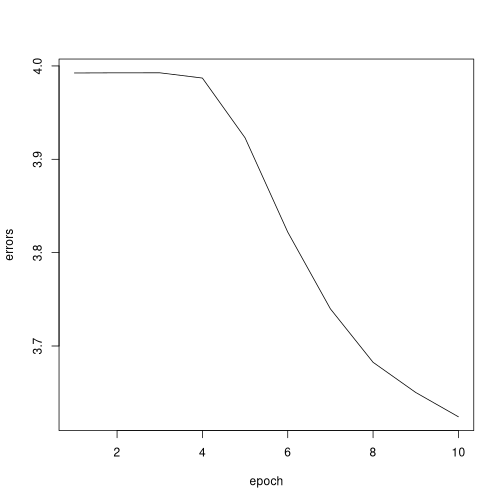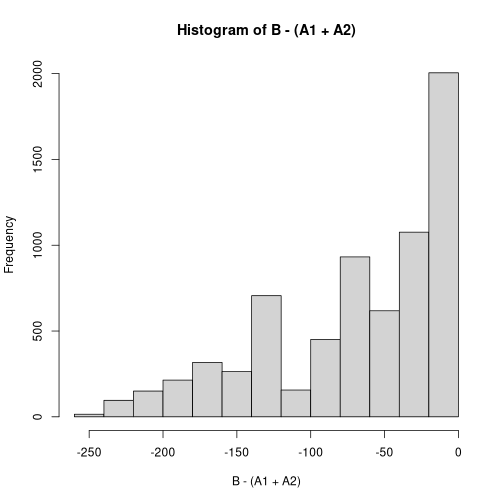# Package

This package includes an example Recurrent Neural Network. The package is loaded using:

``````library(rnn)
``````
``````##
## Attaching package: 'rnn'
``````
``````## The following object is masked _by_ '.GlobalEnv':
##
##     int2bin
``````

# Code

We can view the code of the main `rnn()` function by calling it without the parathesis (not printed here).

``````trainr
``````

As can be seen from the above, the model relies on two other functions that are available through the `sigmoid` package.

The first function is `logistic()`, which converts an integer to its sigmoid value.

``````(a <- sigmoid::logistic(3))
``````
``````##  0.9525741
``````

The code for the `sigmoid()` function is:

``````sigmoid::logistic
``````
``````## function(x, k=1, x0=0)
##   1 / (1+exp( -k*(x-x0) ))
## <bytecode: 0x5591ca659d20>
## <environment: namespace:sigmoid>
``````

The second function converts the sigmoid value of a number to its derivative.

``````sigmoid::sigmoid_output_to_derivative(a) # a was created above using sigmoid()
``````
``````##  0.04517666
``````

Finally, we can inspect this code using:

``````sigmoid::sigmoid_output_to_derivative
``````
``````## function(x)
##   x*(1-x)
## <bytecode: 0x5591ca98a760>
## <environment: namespace:sigmoid>
``````

# Application

An example is included in the help file.

``````help('trainr')
``````

Below is a basic function that converts integers to binary format (read left to right)

``````# basic conversion
i2b <- function(integer, length=8)
as.numeric(intToBits(integer))[1:length]

# apply to entire vectors
int2bin <- function(integer, length=8)
t(sapply(integer, i2b, length=length))
``````

First we generate the data:

``````# create sample inputs
X1 = sample(0:127, 5000, replace=TRUE)
X2 = sample(0:127, 5000, replace=TRUE)

# create sample output
Y <- X1 + X2

# convert to binary
X1 <- int2bin(X1)
X2 <- int2bin(X2)
Y  <- int2bin(Y)

# Create 3d array: dim 1: samples; dim 2: time; dim 3: variables.
X <- array( c(X1,X2), dim=c(dim(X1),2) )
Y <- array( Y, dim=c(dim(Y),1) )
``````

This example is:

``````# train the model
model <- trainr(Y=Y[,dim(Y):1,,drop=F], # we inverse the time dimension
X=X[,dim(X):1,,drop=F], # we inverse the time dimension
learningrate   =  0.1,
hidden_dim     = 10,
batch_size = 100,
numepochs = 10)
``````
``````## Trained epoch: 1 - Learning rate: 0.1
``````
``````## Epoch error: 3.99243809117081
``````
``````## Trained epoch: 2 - Learning rate: 0.1
``````
``````## Epoch error: 3.99261491532439
``````
``````## Trained epoch: 3 - Learning rate: 0.1
``````
``````## Epoch error: 3.99263705025547
``````
``````## Trained epoch: 4 - Learning rate: 0.1
``````
``````## Epoch error: 3.98700261803588
``````
``````## Trained epoch: 5 - Learning rate: 0.1
``````
``````## Epoch error: 3.92298698772648
``````
``````## Trained epoch: 6 - Learning rate: 0.1
``````
``````## Epoch error: 3.82203619961748
``````
``````## Trained epoch: 7 - Learning rate: 0.1
``````
``````## Epoch error: 3.73978583613765
``````
``````## Trained epoch: 8 - Learning rate: 0.1
``````
``````## Epoch error: 3.68253405115345
``````
``````## Trained epoch: 9 - Learning rate: 0.1
``````
``````## Epoch error: 3.65019747135912
``````
``````## Trained epoch: 10 - Learning rate: 0.1
``````
``````## Epoch error: 3.62417332739598
``````

See the evolution of the error over different epochs:

``````plot(colMeans(model\$error),type='l',
xlab='epoch',
ylab='errors'                  )
``````Now create testing data

``````# create test inputs
A1 = int2bin( sample(0:127, 7000, replace=TRUE) )
A2 = int2bin( sample(0:127, 7000, replace=TRUE) )

# create 3d array: dim 1: samples; dim 2: time; dim 3: variables
A <- array( c(A1,A2), dim=c(dim(A1),2) )
``````

Predict based on testing data.

``````# predict
B  <- predictr(model,
A[,dim(A):1,,drop=F]
)
B = B[,dim(B):1]
``````

Define basic functions to convert binary to integer

``````b2i <- function(binary)
packBits(as.raw(c(binary, rep(0, 32-length(binary) ))), 'integer')

bin2int <- function(binary){
binary <- round(binary)
length <- dim(binary)    # determine length of binary representation
apply(binary, 1, b2i)     } # apply to full matrix
``````

Test prediction against true values

``````# convert back to integers
A1 <- bin2int(A1)
A2 <- bin2int(A2)
B  <- bin2int(B)

# plot the difference
hist( B-(A1+A2) )
``````